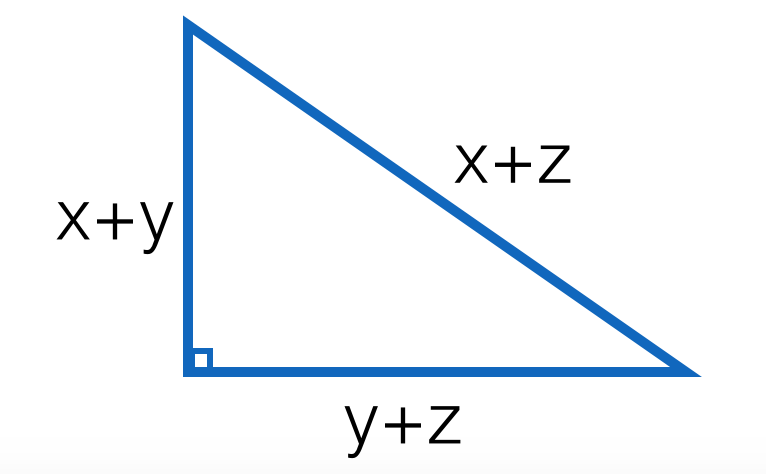# Right Triangle's Variable Sides

Geometry Level 2In a certain right triangle, the hypotenuse equals $(x+z)$ while the other two sides are $(x+y)$ and $(y+z)$ for positive integers $x, y, z$.

If $y+4=z$ and $z>x$ , then compute $\dfrac{z}{x}$.

×